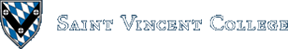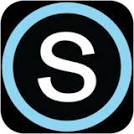< CIS Department > Tutorials > Windows Script Host > Using WSH with an Excel SpreadsheetComputing & Information Systems    DepartmentSearch CIS Site      Tutorials

## Using WSH with an Excel Spreadsheet

The following script shows how WSH can be used to create a new Excel spreadsheet, to place data and formulas in it, to format a cell or cells, and to save the spreadsheet. WSH can be used to create or manipulate a Word document in a similar manner.

 `````` ' Filename: ss.vbs ' ' Author: Br. David Carlson ' ' Date: August 5, 2001 ' ' This WSH script places some data (including a formula) into ' an Excel spreadsheet, formats the spreadsheet, and saves it. Dim xapp Dim workbook Dim worksheet Dim k set xapp = WScript.CreateObject("Excel.Application") xapp.Visible = True ' Make a new Excel workbook: set workbook = xapp.Workbooks.Add set worksheet = workbook.Worksheets("sheet1") for k = 1 to 12 worksheet.Cells(k, 2).Value = 2 * k + 1 next worksheet.Cells(14, 1).Value = "Total:" worksheet.Cells(14, 2).Value = "=sum(B1:B12)" worksheet.Cells(14, 1).Font.Italic = True workbook.SaveAs("C:\sstest.xls") 'Use xapp.Quit if you want to quit Excel. ``````

The script begins by creating an Excel application object. The application is made visible on the screen. You can omit this line if you don't want the application to be seen on the screen. A new workbook is then added. In sheet1, some integer values are placed in cells B1 through B14. Note that worksheet.Cells(14, 2) refers to the cell at row 14, column B. Similarly, worksheet.Cells(5, 3) would refer to the cell at row 5, column C. A formula is placed into a cell as a string starting with an = sign. The particular cell A14 is formatted in italics. SaveAs is used to save the workbook to a particular file. Excel is left running by this script.

Should you want to open an existing workbook, use Open as in the following example:
``xapp.WorkBooks.Open("C:\MySpreadsheet.xls")``
When your script is finished with a workbook, you can close the workbook (but leave Excel running) as in the example below. We assume that `workbook` is set up as in the main example above.
``workbook.Close``
Finally, if you want to quit Excel, use something like the following, where `xapp` is assumed to be set up as in the main example above.
``xapp.Quit``

### More on Using WSH with Office

There is much more that can be done with Windows Script Host to manipulate Office applications and their documents. You can even email an Office document as an attachment! For further information see the References section.

### Back to main Windows Script Host page

Author: Br. David Carlson
Last updated: August 03, 2006
Disclaimer